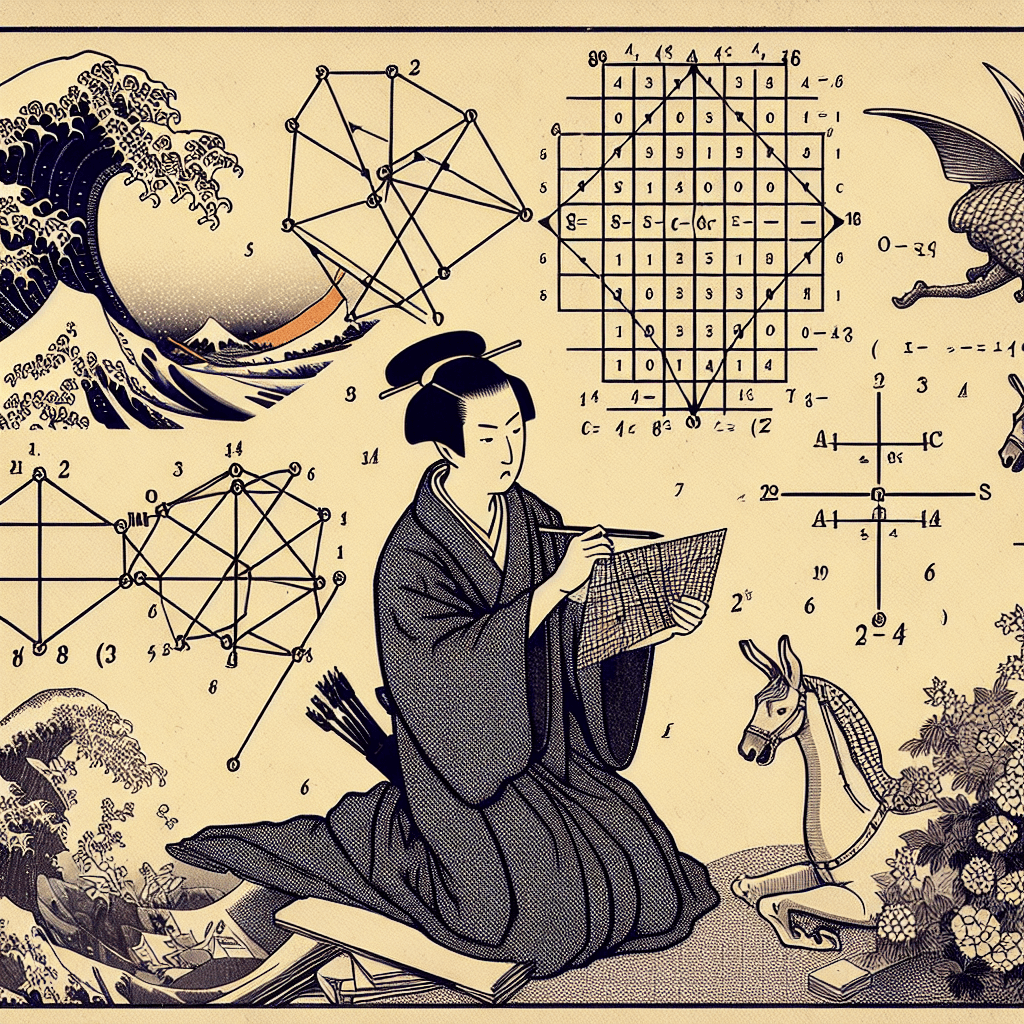# Basics of Linear Algebra: Vectors, Matrices, and Operations

syndu | June 6, 2023, 2:31 p.m.Of course! Let's go over the basics of linear algebra, including vectors, matrices, and common operations.

### Vectors

A vector is a one-dimensional array of numbers. It can be represented as a column or a row. Vectors are often used to represent quantities that have both magnitude and direction, such as velocity or force. Here's an example of a column vector:

``````
v = | 2 |
| 3 |
| 4 |
``````

### Matrices

A matrix is a two-dimensional array of numbers arranged in rows and columns. It can be thought of as a collection of vectors. Here's an example of a 3x3 matrix:

``````
M = | 1  2  3 |
| 4  5  6 |
| 7  8  9 |
``````

### Operations

1. Addition and subtraction: To add or subtract two matrices or vectors, they must have the same dimensions. You add or subtract corresponding elements. For example:

``````
A = | 1  2 |    B = | 4  5 |    A + B = | 1+4  2+5 | = | 5  7 |
| 3  4 |        | 6  7 |            | 3+6  4+7 |   | 9 11 |
``````
2. Scalar multiplication: To multiply a matrix or vector by a scalar (a single number), you multiply each element by the scalar. For example:

``````
c = 2    A = | 1  2 |    cA = | 2*1  2*2 | = | 2  4 |
| 3  4 |         | 2*3  2*4 |   | 6  8 |
``````
3. Matrix multiplication: To multiply two matrices, the number of columns in the first matrix must equal the number of rows in the second matrix. The result is a matrix with the same number of rows as the first matrix and the same number of columns as the second matrix. To compute the elements of the resulting matrix, you perform the dot product of the corresponding row from the first matrix and the corresponding column from the second matrix. For example:

``````
A = | 1  2 |    B = | 4  5 |    AB = | (1*4 + 2*6)  (1*5 + 2*7) | = | 16  19 |
| 3  4 |        | 6  7 |         | (3*4 + 4*6)  (3*5 + 4*7) |   | 36  43 |
``````
4. Matrix inversion: The inverse of a square matrix (a matrix with the same number of rows and columns) is a matrix that, when multiplied by the original matrix, results in the identity matrix (a matrix with 1s on the diagonal and 0s elsewhere). Not all matrices have inverses; a matrix must be non-singular (i.e., its determinant is non-zero) to have an inverse. Inverting a matrix can be done using various methods, such as Gaussian elimination or the adjugate method. The inverse of a 2x2 matrix can be computed as follows:

``````
A = | a  b |    A_inv = 1/det(A) * |  d -b |
| c  d |                        | -c  a |

where det(A) = ad - bc (the determinant of A)
``````

These are the basics of linear algebra. As you delve deeper into the subject, you'll encounter more advanced concepts and operations, such as eigenvectors, eigenvalues, and matrix decompositions. However, the fundamentals outlined here will serve as a solid foundation for your further studies.

##### Lilith's Grimoire

The app you are using right now is Lilith's Grimoire

The Grimoire is full of Source Code. It is alive, and running, and you can explore the way it is built

The first lesson uncovers all of it.#### Unraveling Matrices: An In-Depth Look at Cofactor Expansion#### Week 4: Eigenvalues - Unveiling the Hidden Power of Matrices#### Understanding Matrix Determinants, Cofactor Expansion, and Systems of Linear Equations#### Understanding and Calculating Determinants#### Mastering Matrices: An Overview of Their Properties and Operations#### Mastering the Basics: An Introduction to Vectors#### Vector Spaces: The Foundation of High-Dimensional Data in Language Models#### Crucial Linear Algebra Topics in Large Language Models